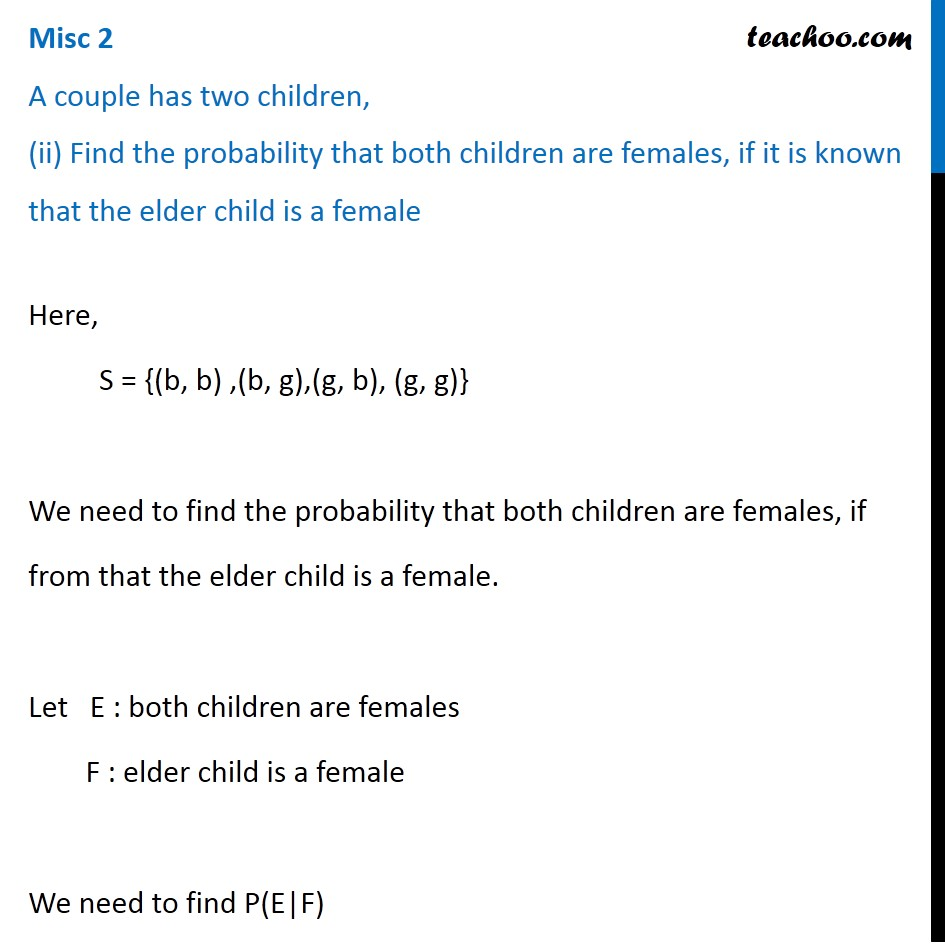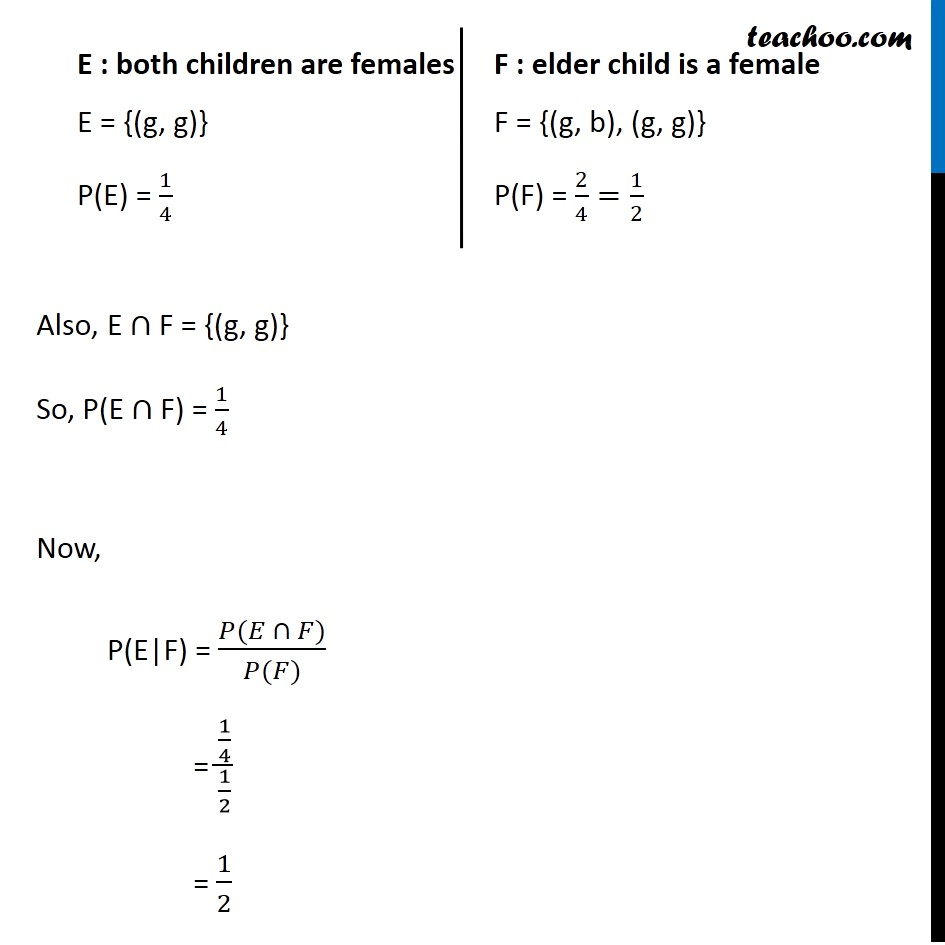Miscellaneous

Chapter 13 Class 12 Probability
Serial order wiseLearn in your speed, with individual attention - Teachoo Maths 1-on-1 Class

### Transcript

Misc 2 A couple has two children, (ii) Find the probability that both children are females, if it is known that the elder child is a femaleHere, S = {(b, b) ,(b, g),(g, b), (g, g)} A We need to find the probability that both children are females, if from that the elder child is a female. Let E : both children are females F : elder child is a female We need to find P(E|F) E : both children are females E = {(g, g)} P(E) = 1/4 F : elder child is a female F = {(g, b), (g, g)} P(F) = 2/4=1/2 Also, E "∩" F = {(g, g)} So, P(E "∩" F) = 1/4 Now, P(E|F) = (𝑃(𝐸 ∩ 𝐹))/(𝑃(𝐹)) = (1/4)/(1/2) = 1/2